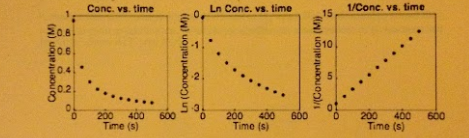# Problem: The three plots shown were graphed using experimental data for the reaction shown below. Based on the graphs, what is the order of the reaction? 3 A + B → 2 C a. zero order b. first order c. second order d. third order e. there is insufficient data to determine the reaction order

###### Problem Details

The three plots shown were graphed using experimental data for the reaction shown below. Based on the graphs, what is the order of the reaction?

3 A + B → 2 C

a. zero order

b. first order

c. second order

d. third order

e. there is insufficient data to determine the reaction order